# NCERT Solutions for Class 10 Maths Chapter 12- Areas Related to Circles

NCERT Solutions for Class 10 Maths Chapter 12- Areas Related to Circles

This chapter contains the solutions to three exercises of NCERT-Textbook, i.e., 12.1, 12.2, and 12.3. All three practiced exercises include answering questions related to Chapter 12, i.e., Area Related to Circles. In this chapter, students are given the solutions to many questions to perform well in CBSE 2023 examinations.

The solutions to the NCERT-based questions of Chapter 12 for Class 10 Maths, Areas Related to Circles, is an essential study resource for Class 10 students. These assist students in comprehending or solving the sorts of problems that a paper setter asked in the 2023 examinations of CBSE Class 10 Maths. Furthermore, offering solutions to all areas relating to circles assists students in effectively preparing for the CBSE 2023 examinations.

NCERT Solutions for Class 10 Maths Chapter 12- Areas Related to Circles | Free PDF Download

The subject matter experts make sure that the errors in the NCERT textbooks are resolved according to the NCERT rules and syllabus.

The solutions to the NCERT-based questions of Chapter 12 for Grade 10 Maths are accessible at our website. These solutions will assist students in acing their examinations by preparing for the examinations ahead of time. Students may clarify all of their conceptual questions or doubts with the assistance of the notes of Maths NCERT Solutions for Class 10 of this chapter which we provide. Experts have created these NCERT Solutions based on the most recent update to the CBSE syllabus for 2022-2023 to help students in Class 10 prepare well for their examinations.

The Class 10 Maths Chapter 12 of NCERT Solutions includes topics related to circles, i.e., circumference and the area of a circle, and how to find the areas of two specific 'sections' of a circular region called sector and segment.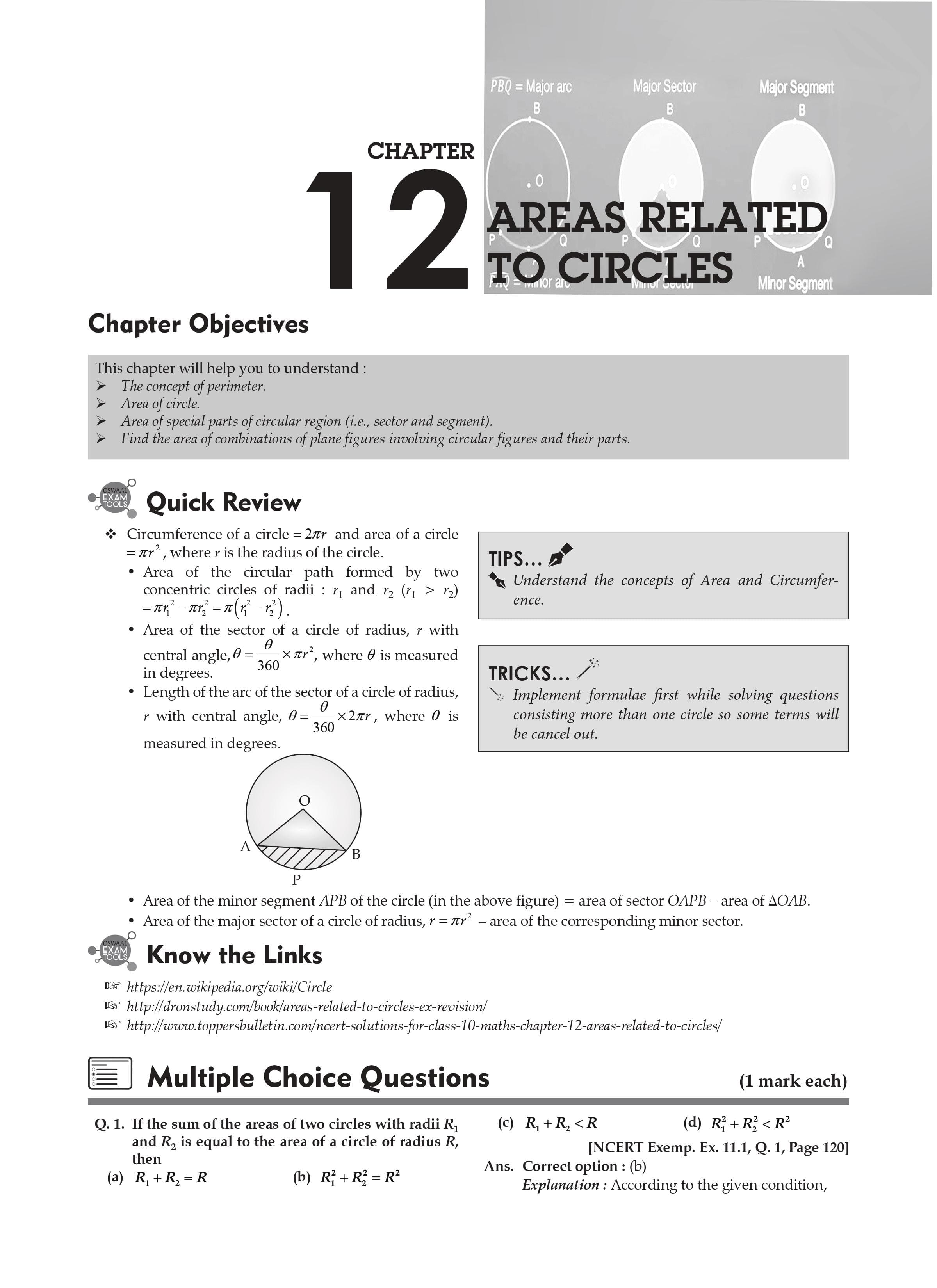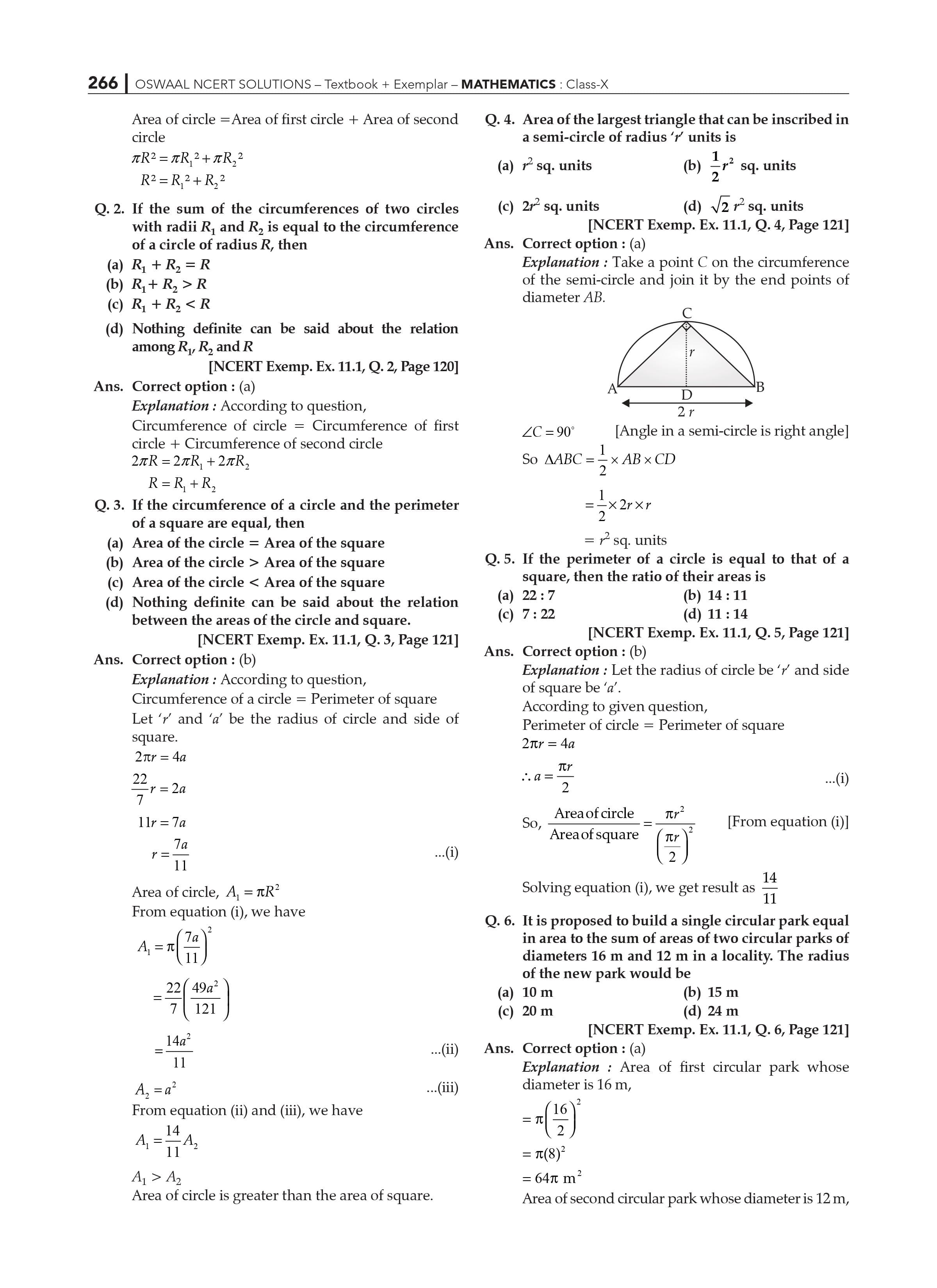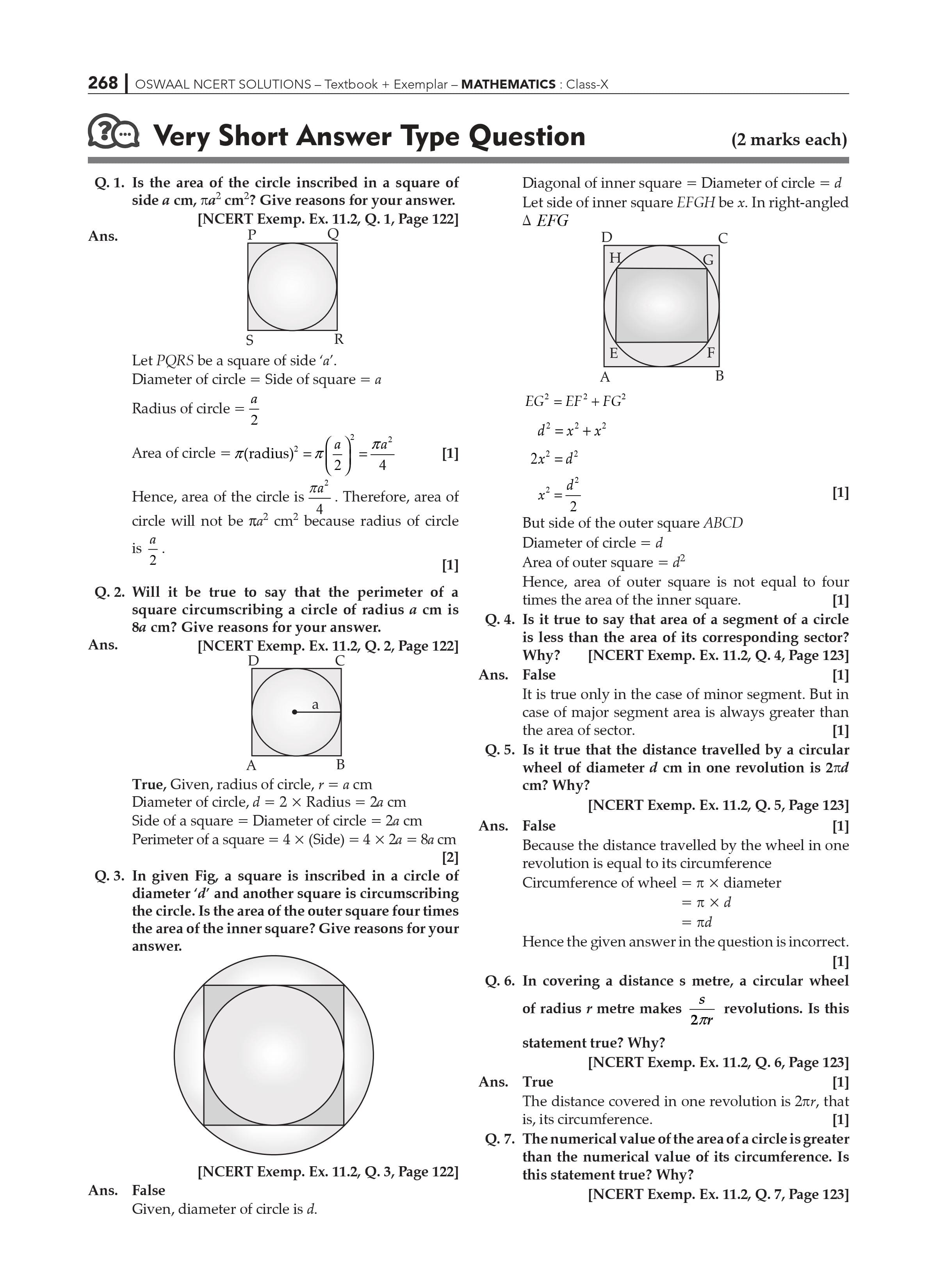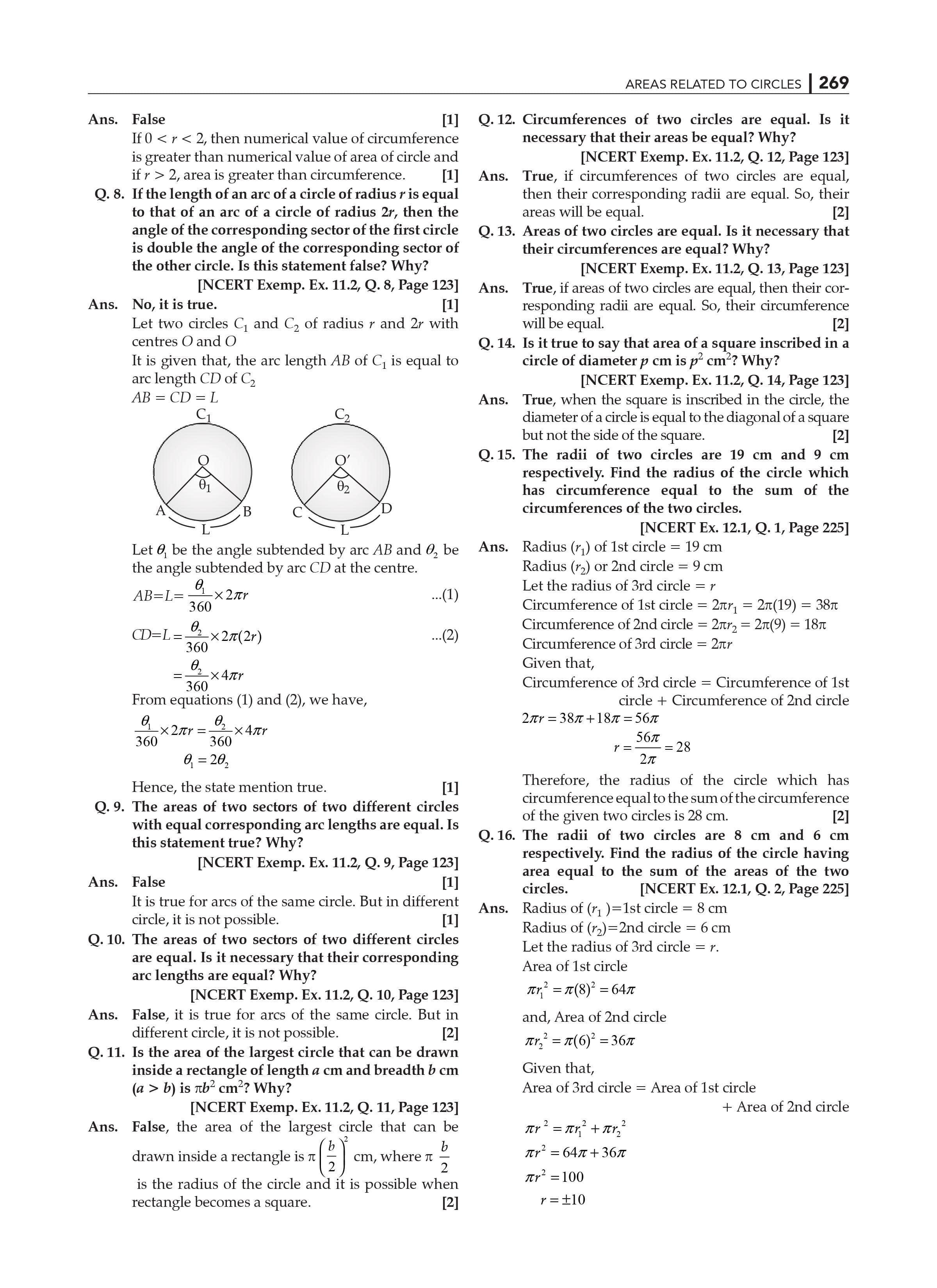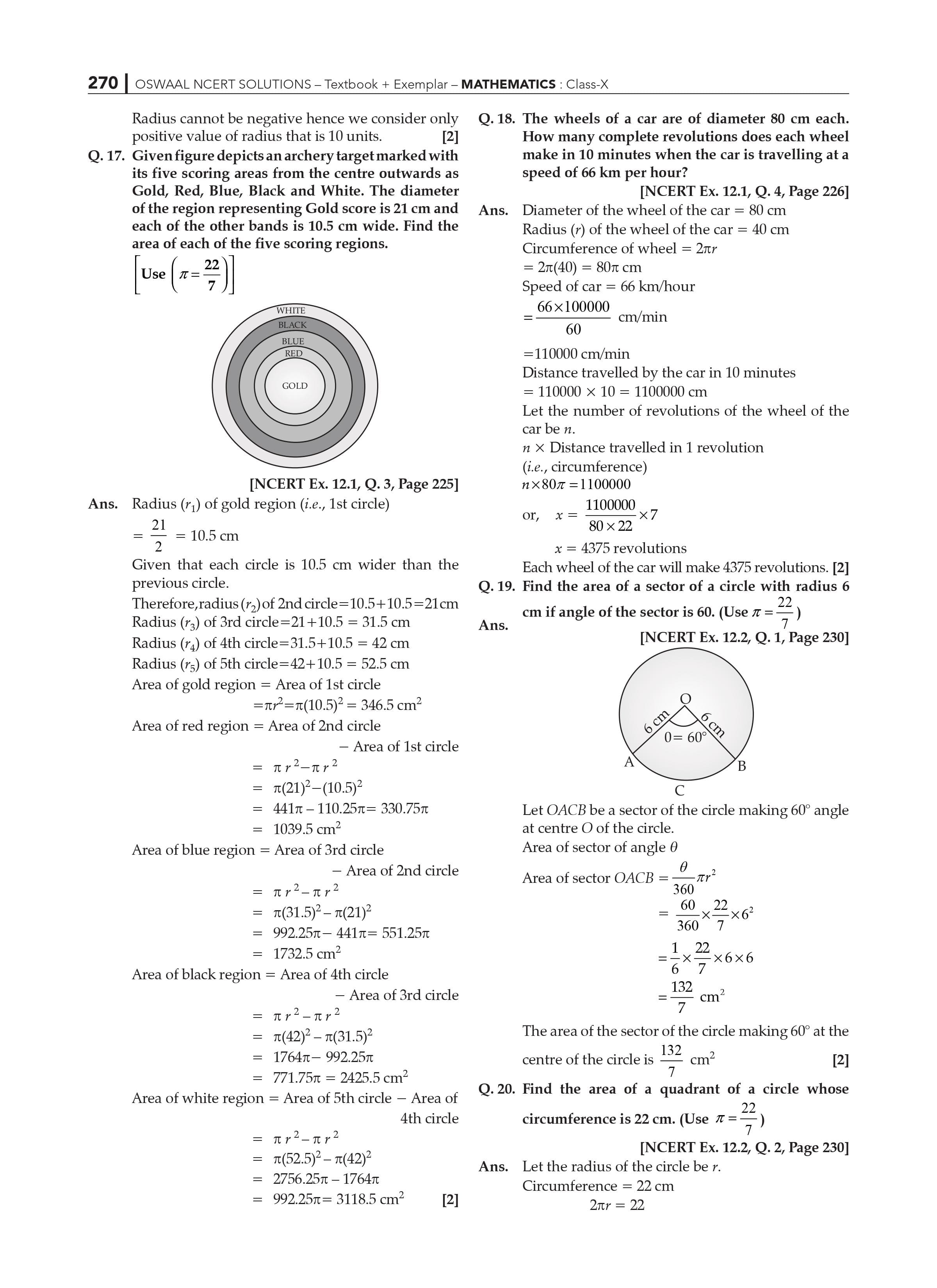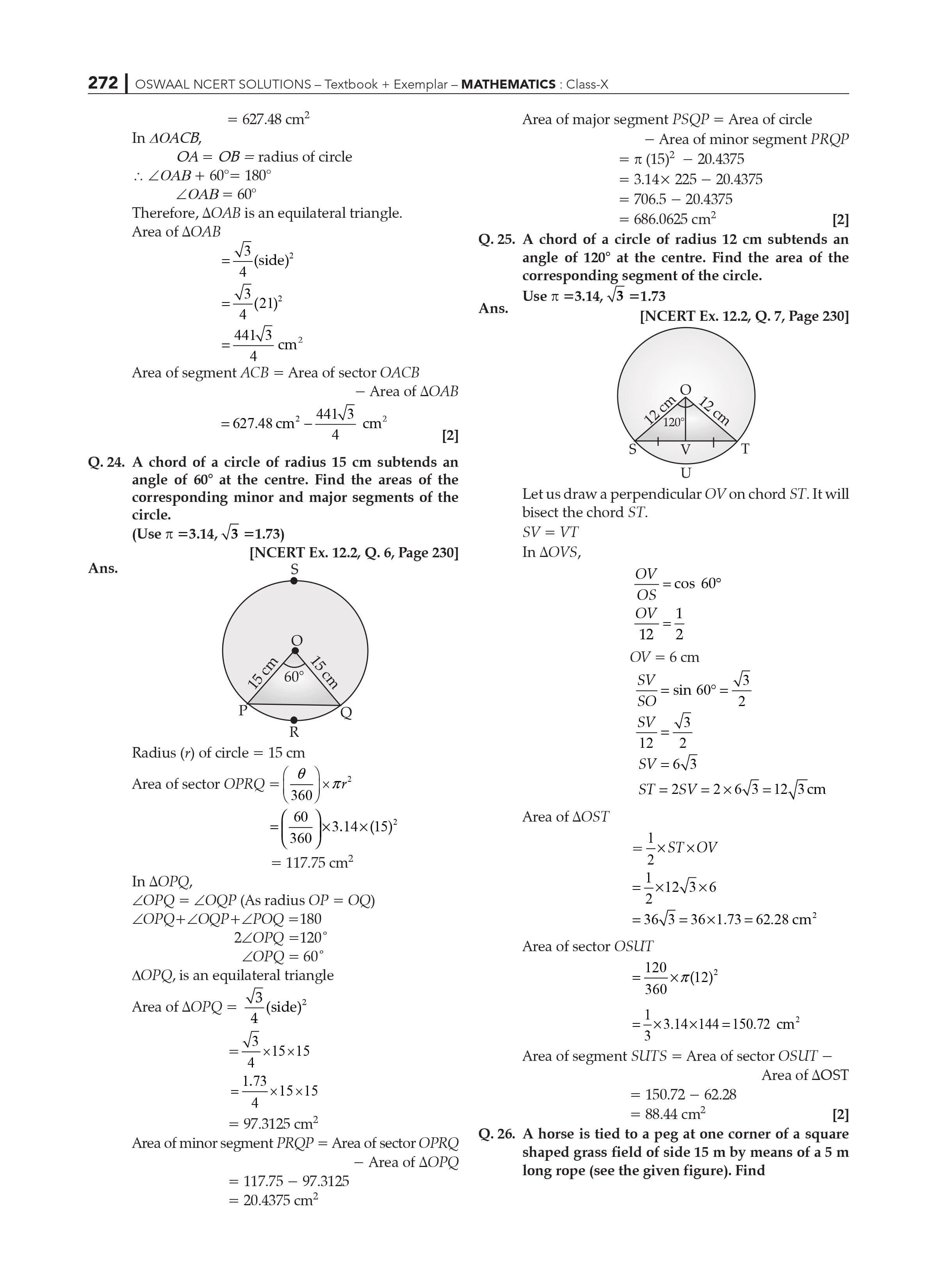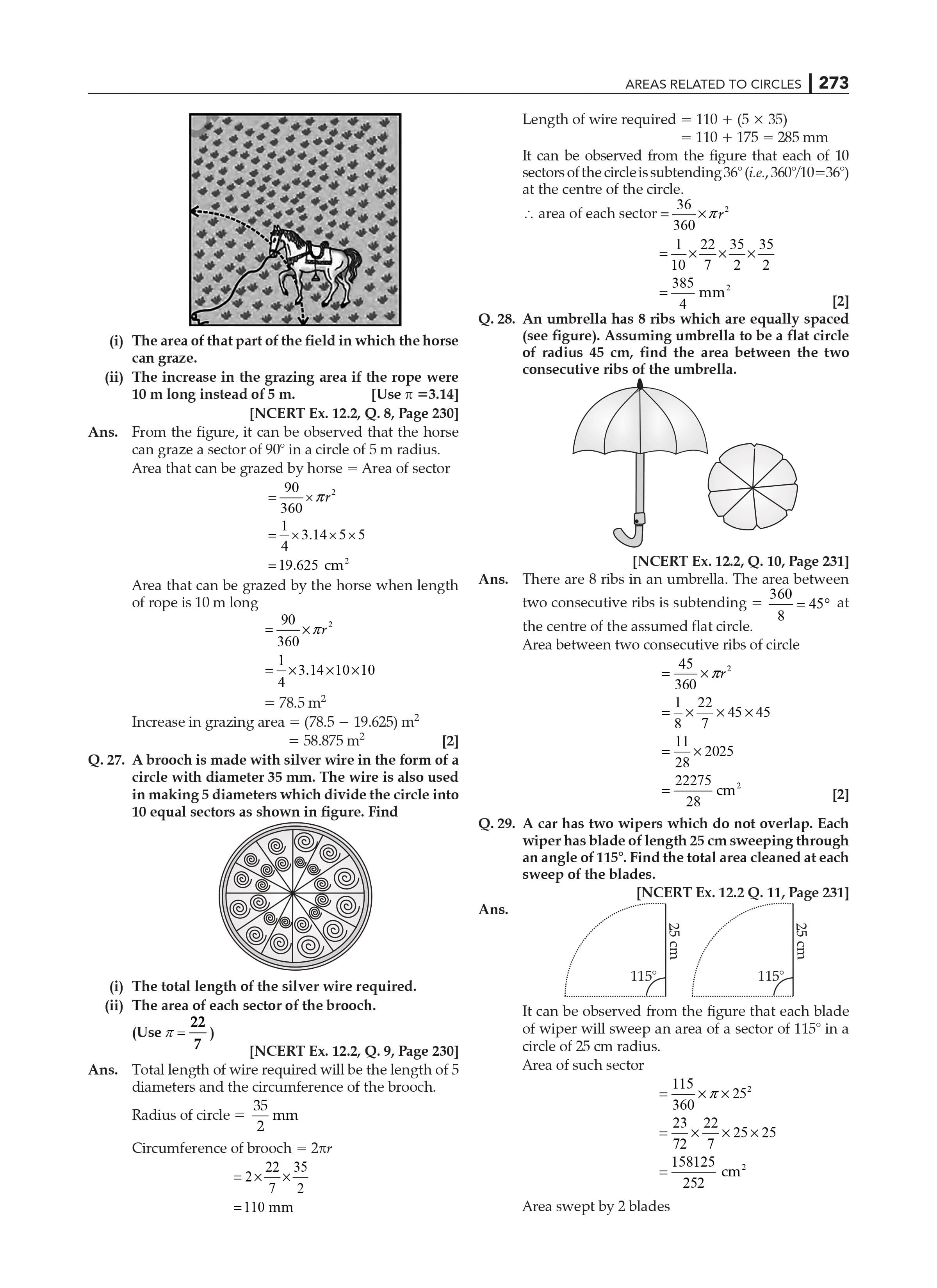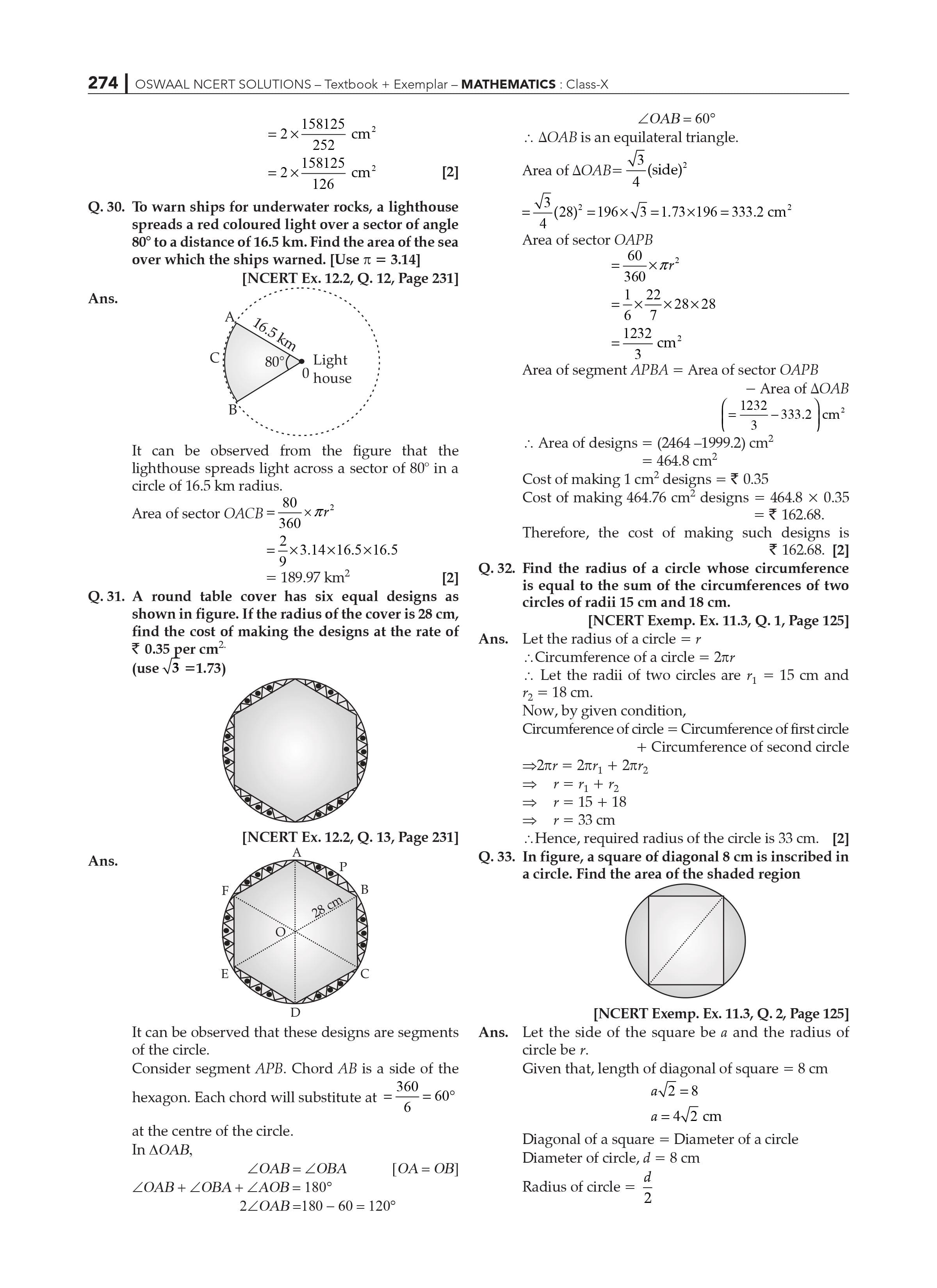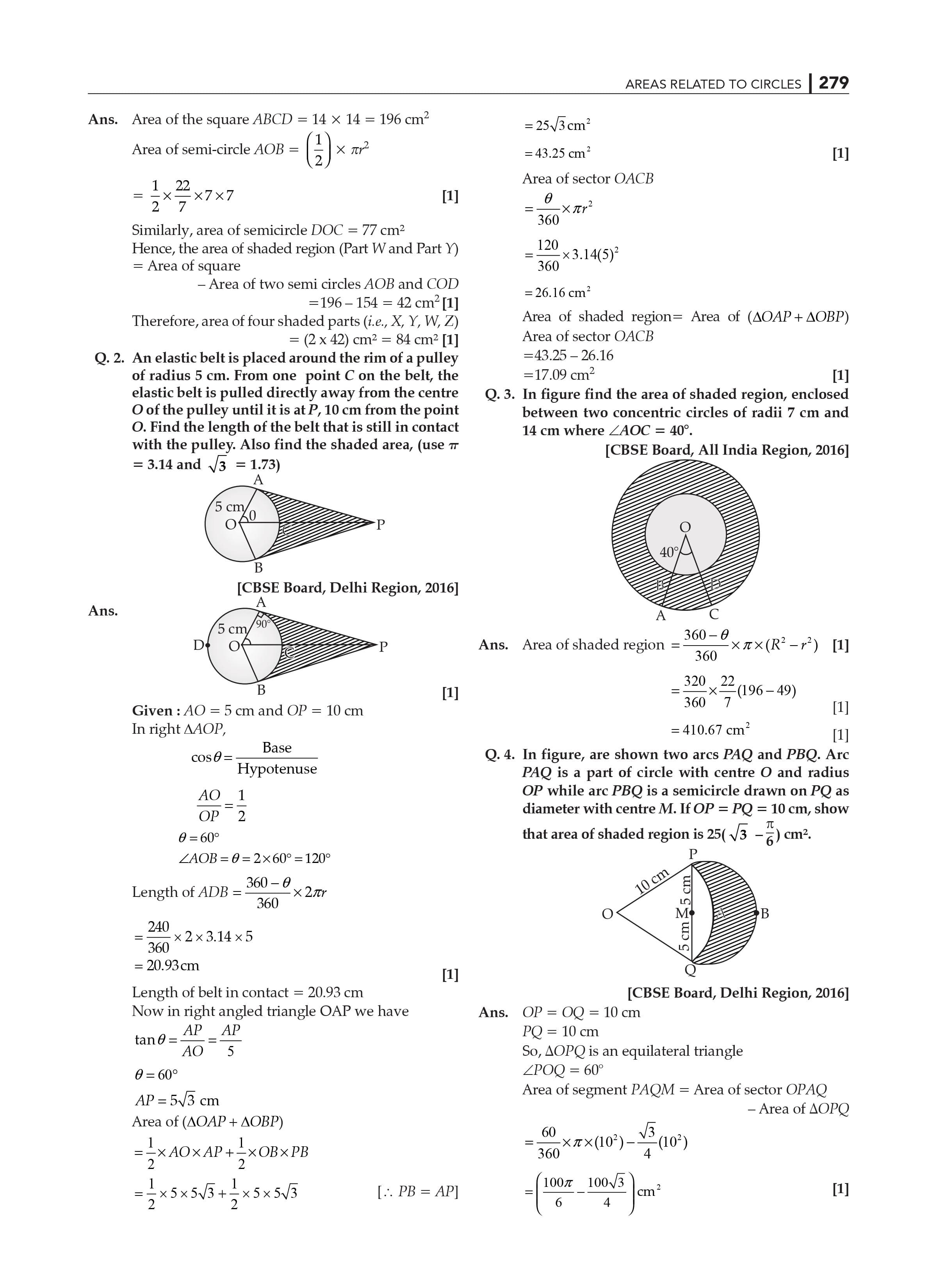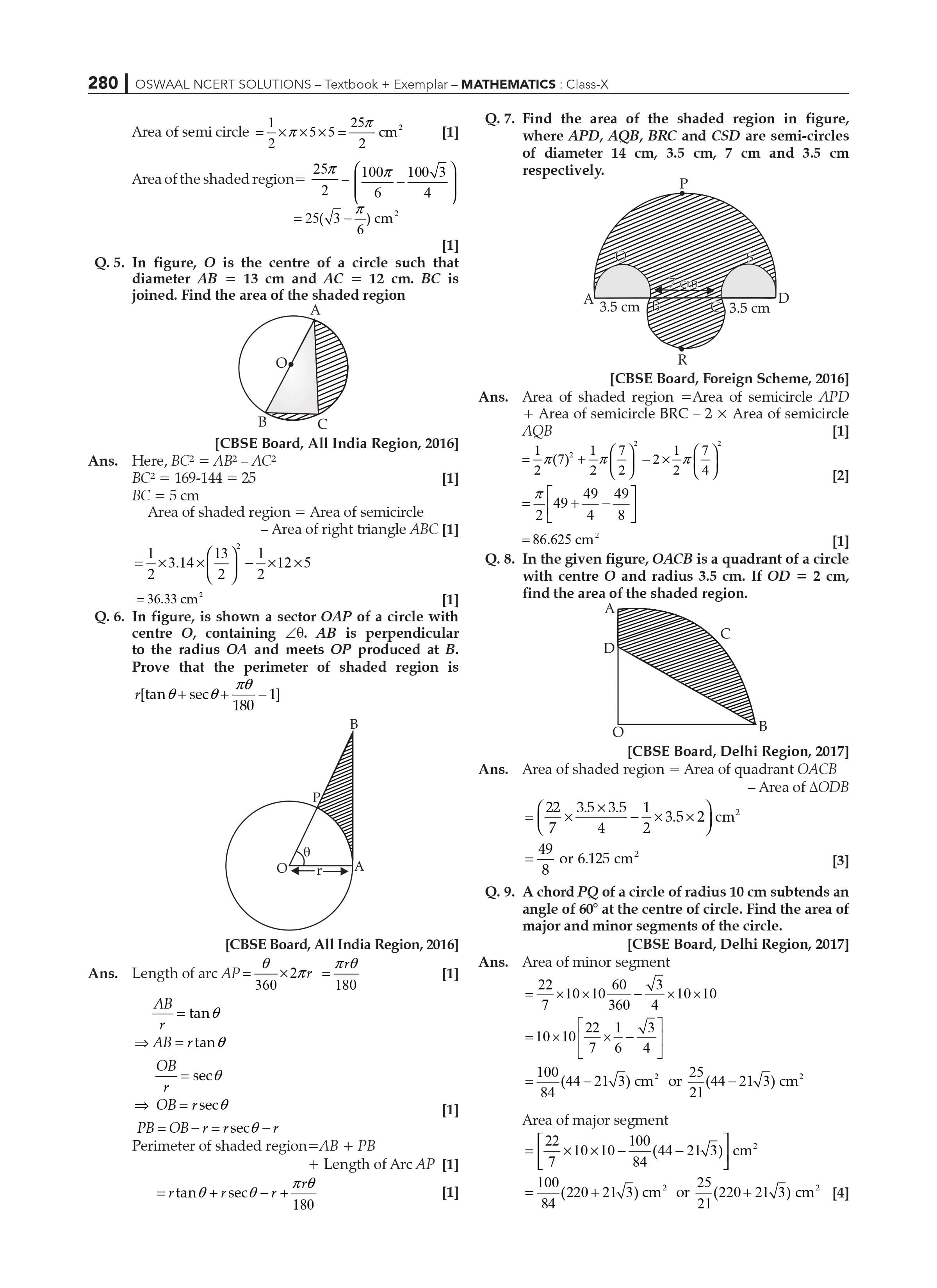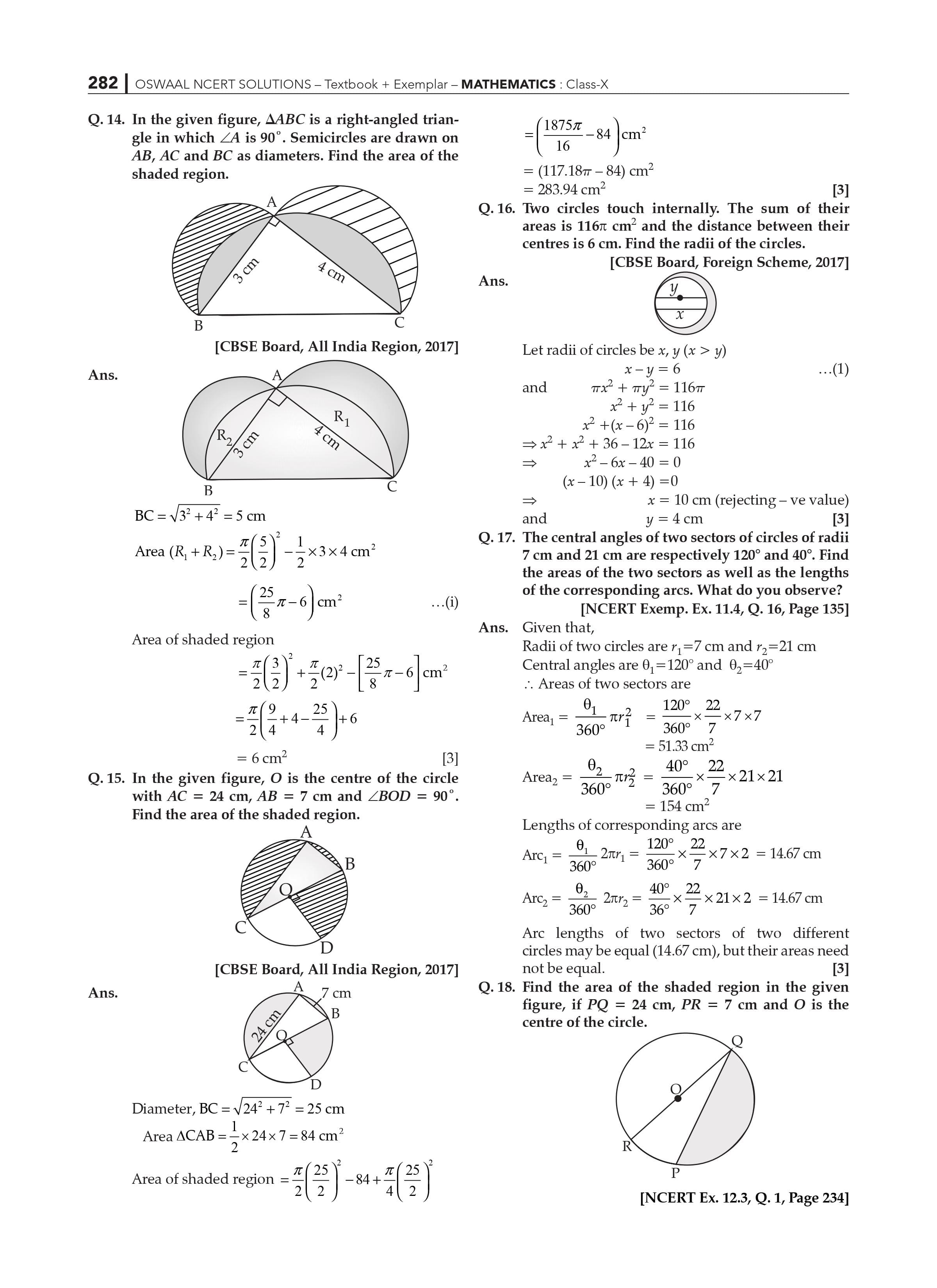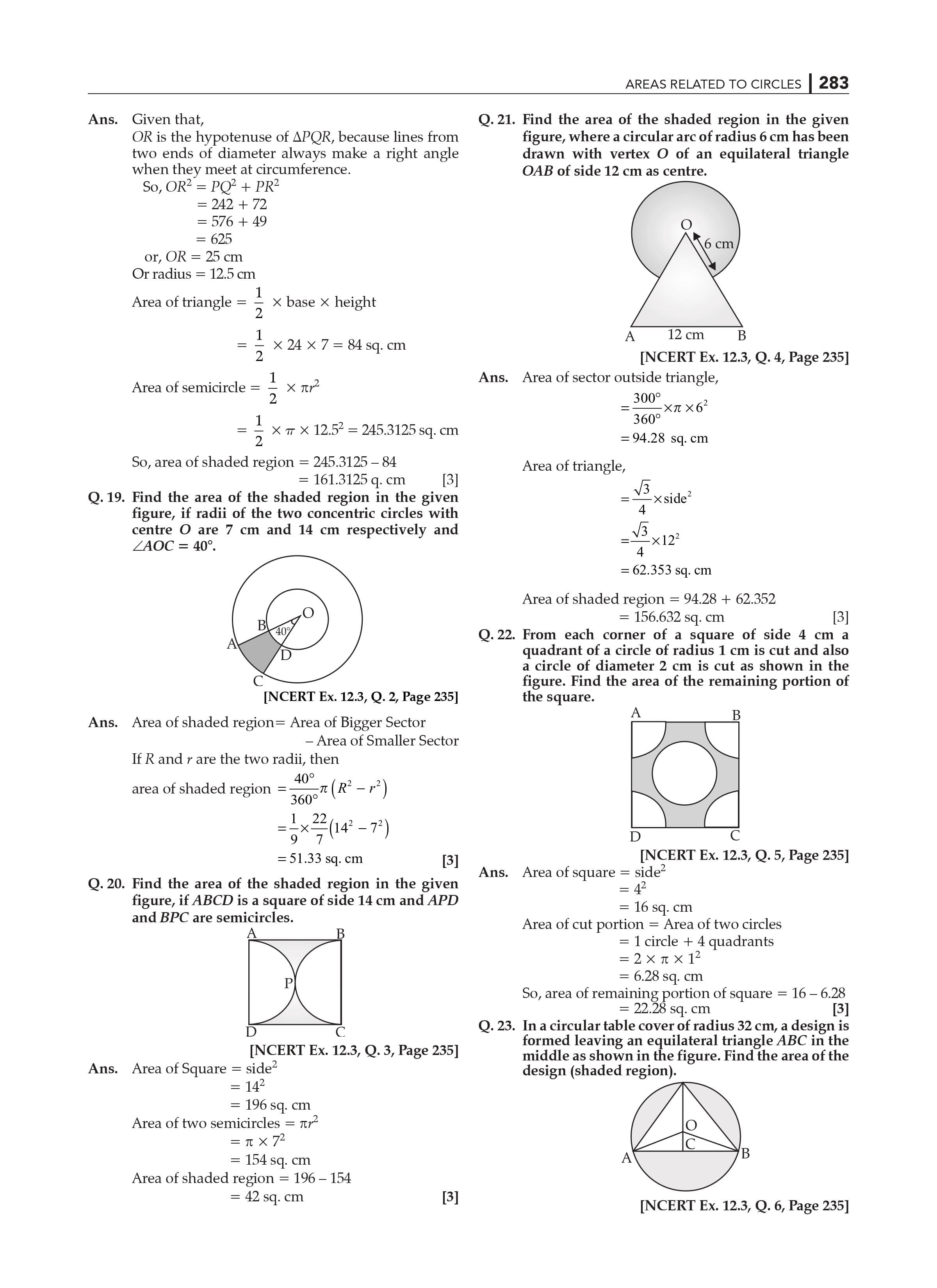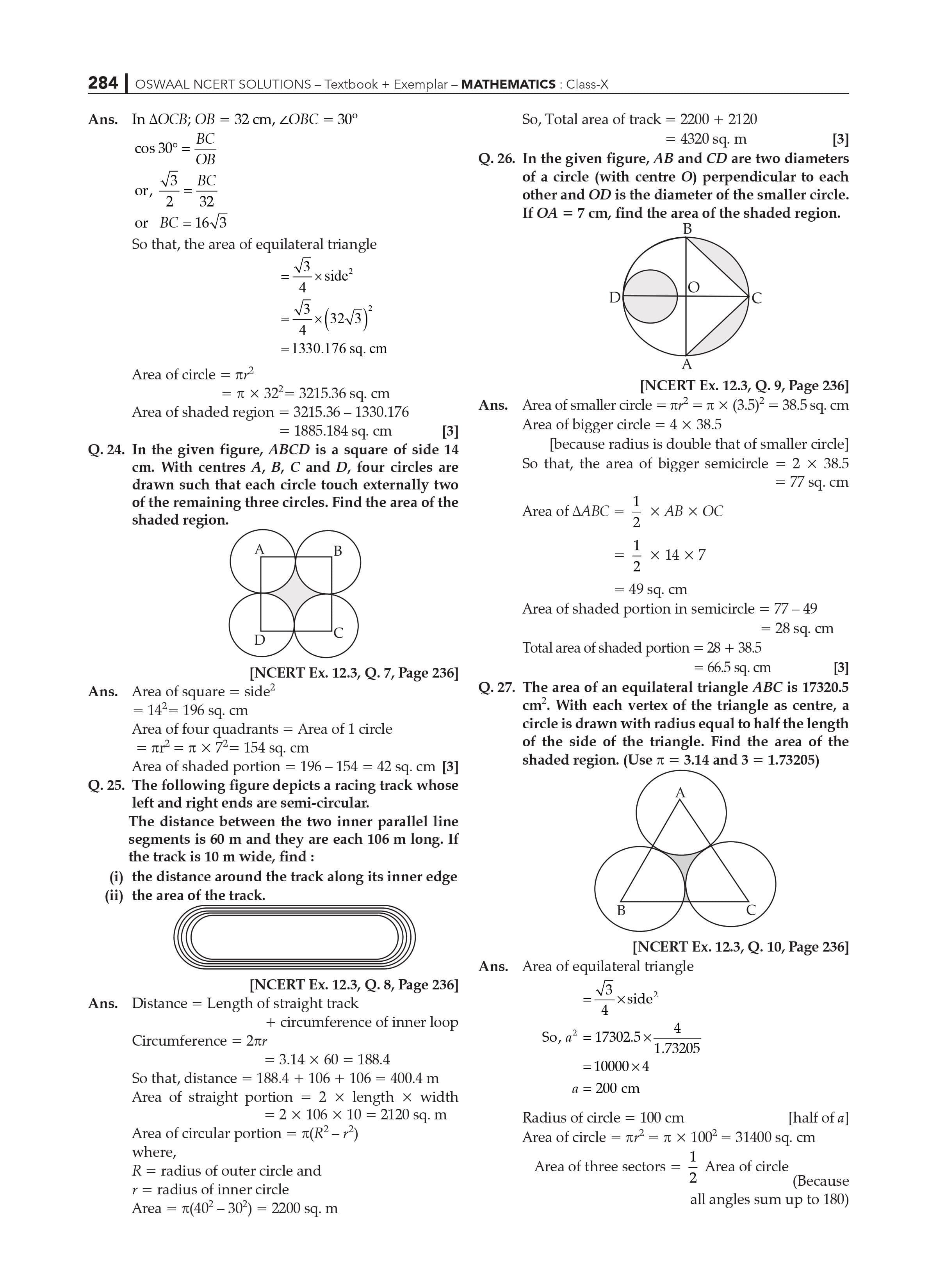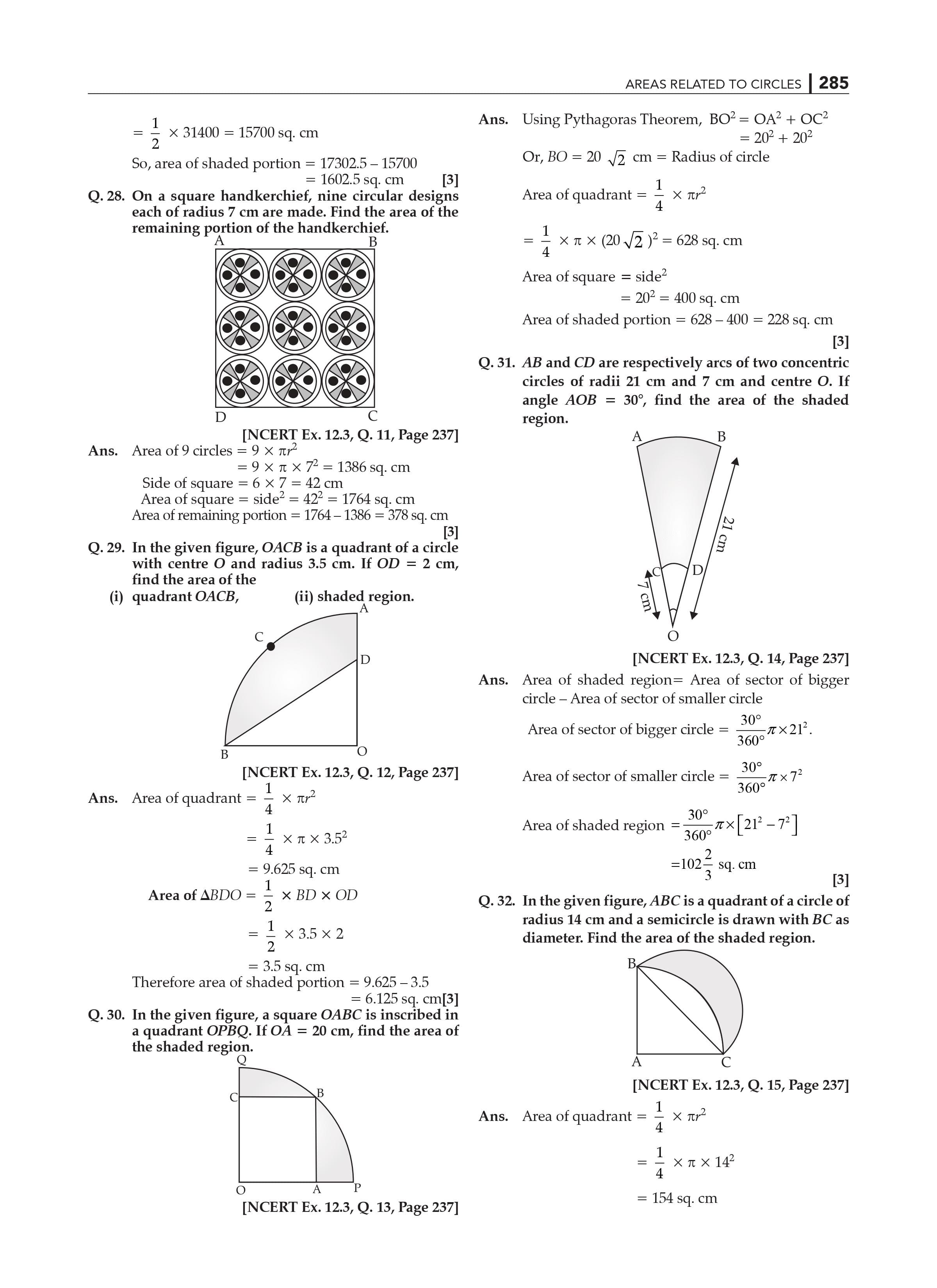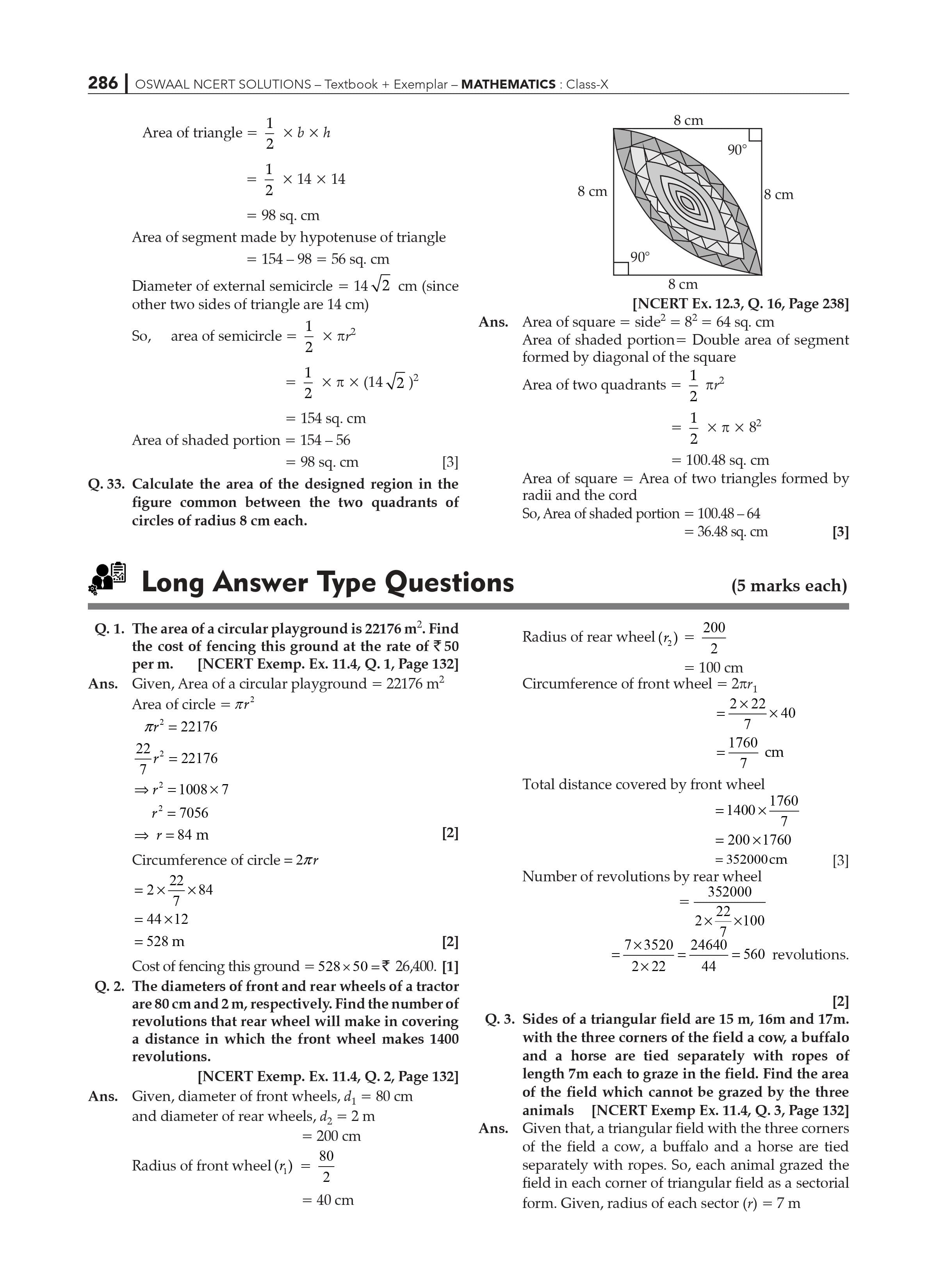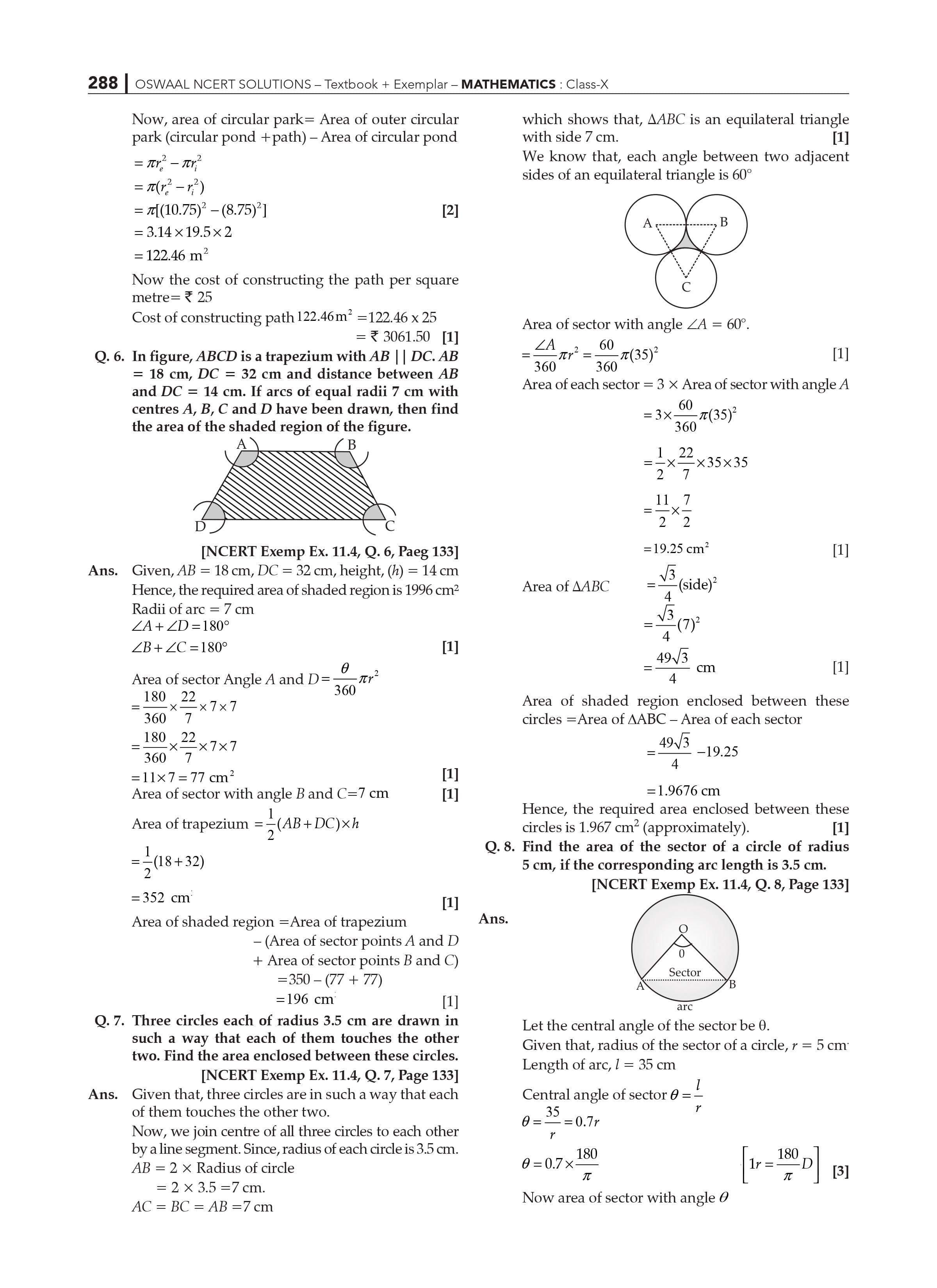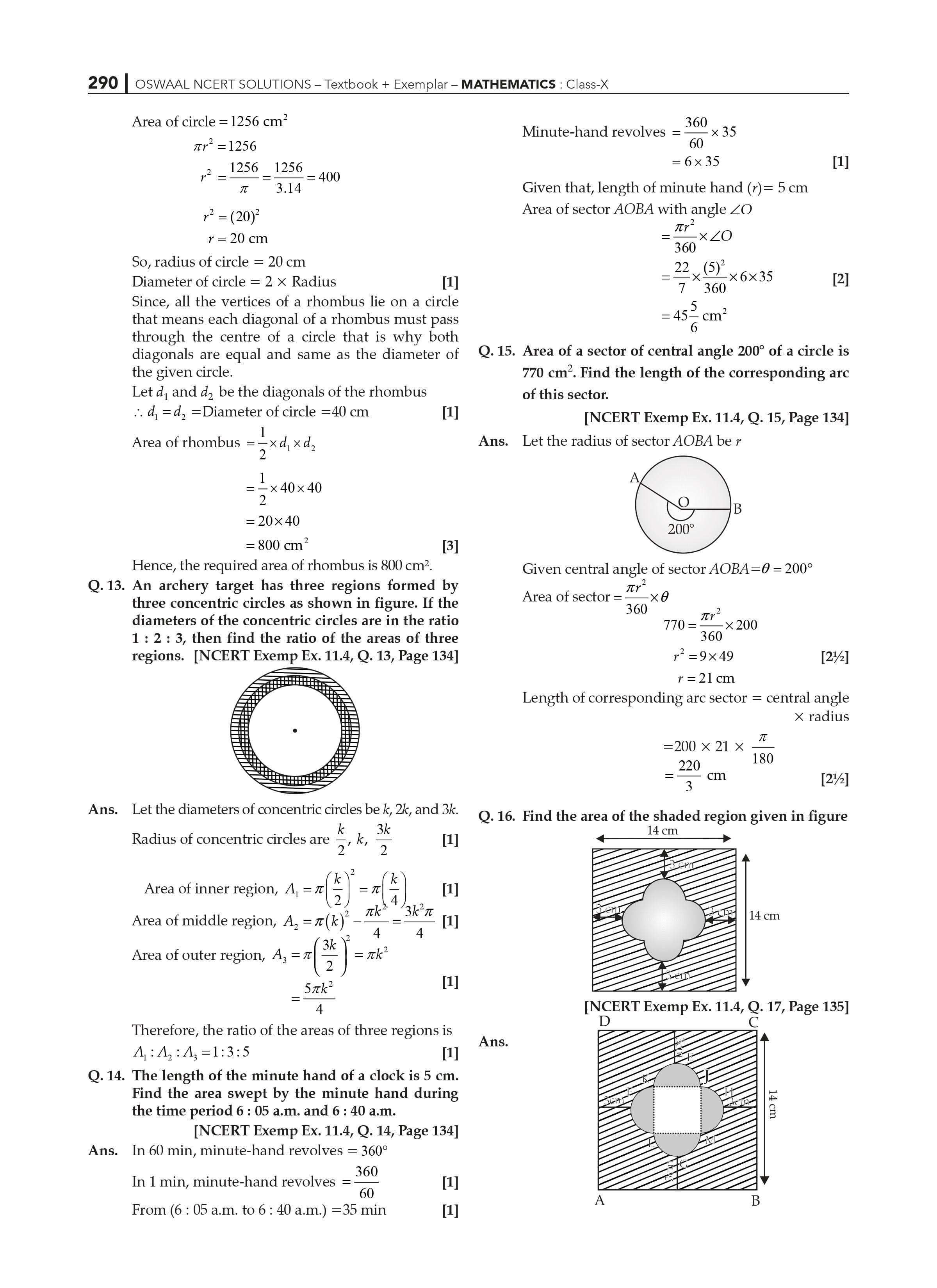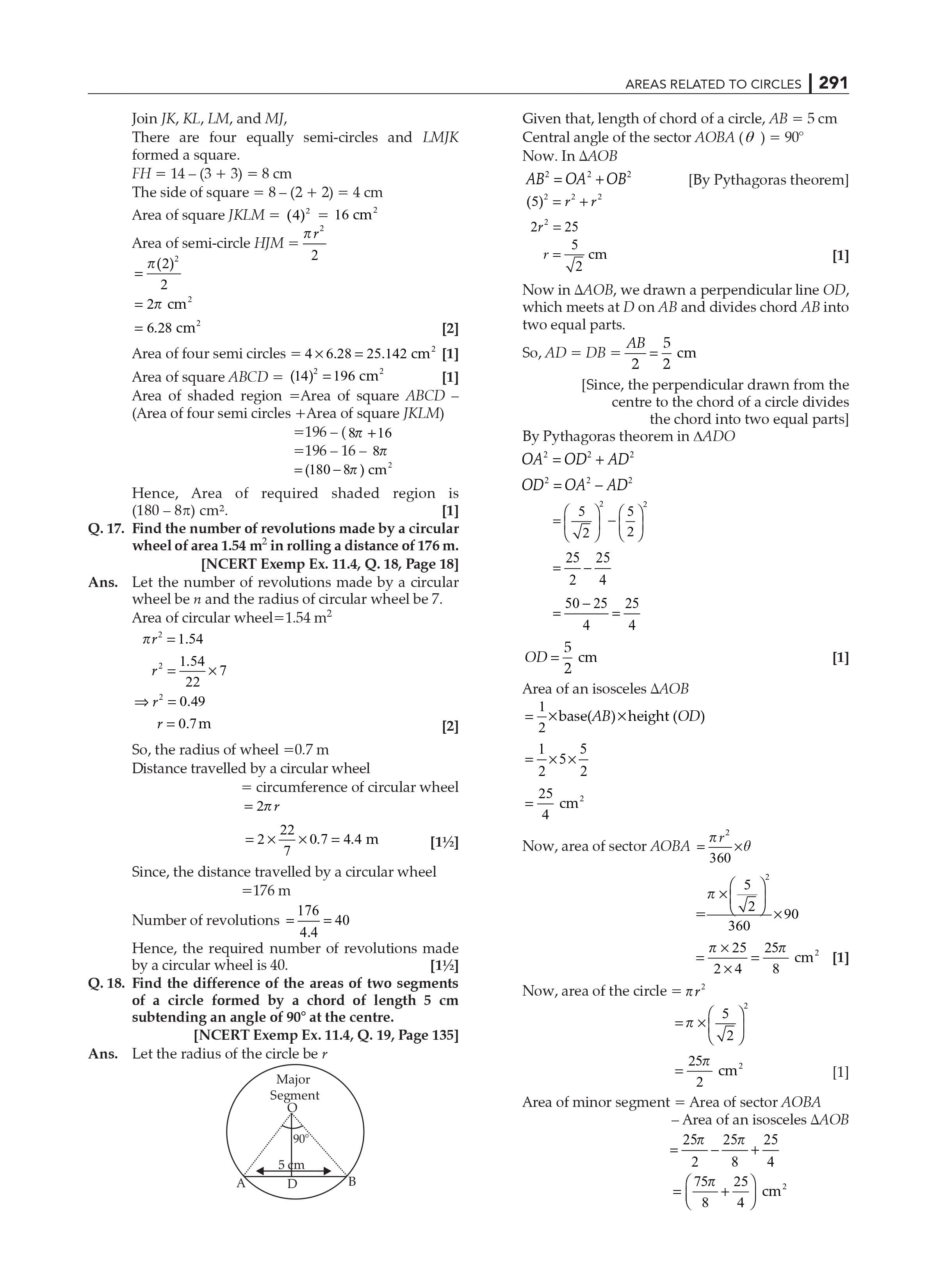Chapter 12 Areas Related to Circles is one of the parts of the Mensuration, and it has a total weightage of 10 points in the examinations. One question from this chapter is frequently asked in the test.

Parts of circles, their measurements, and the areas of planar figures are covered in Chapter 12 of Maths NCERT Solutions for Class 10. Our subject matter specialists have created solutions for each question based on the CBSE syllabus for (2022-23).

 Extra Information: NCERT Exemplar for Class 10 Maths Chapter 12 CBSE Notes for Class 10 Maths Chapter 12

The following are some of the concepts covered in Chapter 12, Areas Related to The Circles are:

Circumference of a circle: The circumference of a circle is the distance around the circle's exterior. It is similar to the perimeter of other forms such as squares. Consider it to be the line that defines the geometry or shape. This defining line is the perimeter for objects with straight edges but the circumference for circles.

Area of Circle: The area of a circle having a radius r is equal to the πr2.

Class 10 Maths NCERT Chapter 12 Areas related to circles include major subjects as:

Exercise 12.1 Introduction

Exercise 12.2 Concept of perimeter and area of a circle

Exercise 12.3 Concept of Areas of Sector and Segment of a Circle

Exercise 12.4 Concept of Areas of Combinations of Plane Figures

Exercise 12.5 Summary

Practicing the NCERT Solutions for Class 10 might be highly important for the CBSE 2023 examinations.

Class 10 Maths NCERT Solutions of Chapter 12 Areas Related to Circles Highlights:

NCERT Solutions assist students in solidifying their knowledge in circle-related areas.

Diagrams are used to answer questions, making learning more dynamic and thorough.

The language used in NCERT Solutions is simple and easy to grasp.

Students benefit from a step-by-step approach to problem-solving.

Allows learners to work through challenging problems in their own time.

NCERT Solutions for various courses and disciplines are also available for students to use. Our well-experienced professors create these answers to offer clarity on essential ideas and problem-solving abilities.

Students can also obtain a firm grasp of critical topics by referring to additional study resources available in our CBSE Class 10 books.

Along with the NCERT Solutions, college students also can exercise the alternative textbook answers to apprehend the ideas in a miles higher way.

Frequently Asked Questions for CBSE Class 10 Maths Chapter 12:

1. What are the benefits of studying NCERT Solutions for Class 10 Maths Chapter 12?

Answer: NCERT Class 10 Maths Chapter 12 solutions assist students in obtaining a summary of the question paper format, which includes a range of questions, such as usually repeated questions, brief and long response type questions, multiple-choice questions, marks, and so on. The more problems you solve, the more confident you will be in your achievement.

1. Mention exam-relevant concepts in NCERT Solutions for Class 10 Maths Chapter 12?

Answer: The most important exam topics in NCERT Solutions for Class 10 Maths Chapter 12 are Introduction to the area of a circle, perimeter and area of a circle, areas of a sector and segment of a circle, areas of combinations of plane figures, and finally a summary of the entire chapter.

1. What number of exercises in NCERT Solutions for Class 10 Maths Chapter 12?

Answer: It includes three exercises. The first exercise has five questions. The second contains 14 questions. The third exercise contains 16 questions based on the perimeter and area of a circle, the areas of a sector and segment of a circle, and the areas of combinations of planar figures, respectively.